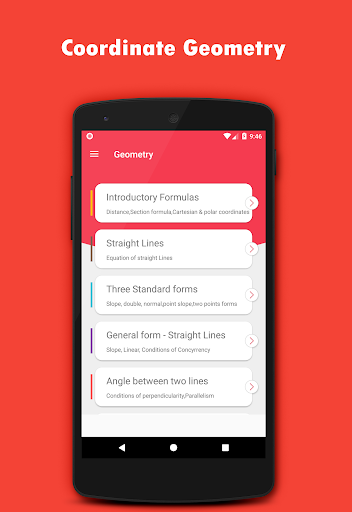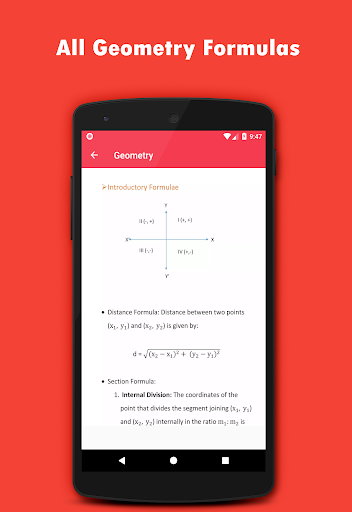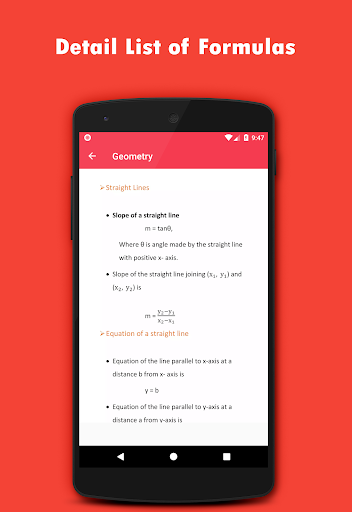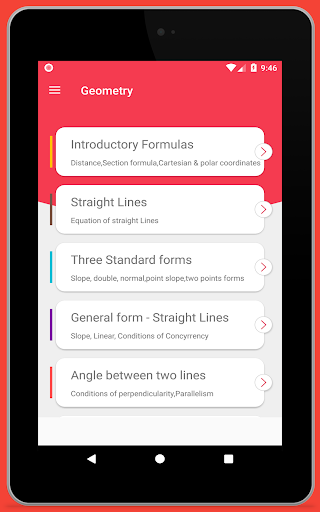This app provides all geometry formulas which is helpful for all level of students and others who require mathematics in there daily life.

Contents :-

1. Introductory Formulas
2. Area of triangle and Cartesian and polar
3. Straight lines
4. Slope, Intercept , Normal form,two point form
5 . General form straight lines
6. Angle between two lines
7. Length of perpendicular, angle bisectors
8. pair of lines
9. Circle
10. equation of tangent and normal
11. conic section- eccentricity
12. Parabola tangent and normal
13. Ellipse
14.Co- ordinates in space
15. Direction cosines and ratios
16. Projection
17. Plane and angle between two planes
All Coordinate geometry formulas in one app